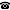# 2014 seminar talk: Algorithmic Randomness and the Turing Degrees

Talk held by Dan Turetsky (KGRC) at the KGRC seminar on 2014-03-27.

### Abstract

Algorithmic randomness uses the tools of computability theory to analyze the question, "When is an infinite binary sequence random?".  Several different definitions of a random sequence are studied, with the most common being Martin-Löf randomness.

Randomness has a rich interaction with the Turing degrees of more classical computability theory, in particular with the c.e. degrees and the PA degrees. For example, if a random cannot compute $0'$ (or equivalently, cannot compute a PA degree), then every c.e. set which it can compute is $K$-trivial, which means that randomness considers it useless as an oracle; despite being non-computable, it has no derandomization power. The covering problem asks if this can be reversed; is every $K$-trivial computable from a random that does not compute $0'$?

I will review the appropriate definitions and discuss the covering problem and similar questions. I will also discuss the proof of the covering problem, which touches on the interaction of algorithmic randomness and effective analysis.

Kurt Gödel Research Center for Mathematical Logic. Währinger Straße 25, 1090 Wien, Austria.+43-1-4277-50501. Last updated: 2010-12-16, 04:37.